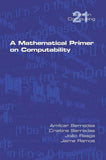•# A Mathematical Primer on Computability

College Publications
SKU: 9781848902961
20 In Stock

Quick Overview

The book provides a self-contained introduction to computability theory for advanced undergraduate or early graduate students of mathematics and computer science. The technical material is illustrated with plenty of examples, problems with fully worked solutions as well as a range of proposed exercises. Part I is centered around fundamental computability...
Regular Price \$26.08 Sale Price Unit price: \$0.00

Secured and trusted checkout with

The book provides a self-contained introduction to computability theory for advanced undergraduate or early graduate students of mathematics and computer science. The technical material is illustrated with plenty of examples, problems with fully worked solutions as well as a range of proposed exercises. Part I is centered around fundamental computability notions and results, starting with the pillar concepts of computational model (an abstract high-level programming language), computable function, decidable and listable set, proper universal function, decision problem and the reduction technique for transferring decidability and listability properties. The essential results namely Rice's Theorem, Rice-Shapiro's Theorem, Rice-Shapiro-McNaughton-Myhill's Theorem as well as Rogers' Theorem and the Recursion Theorem are presented and illustrated. Many-to-one reducibility and many-to-one degrees are investigated. A short introduction to computation with oracles is also included. Computable as well as non-computable operators are introduced as well as monotonic and finitary operators. The relationship between them is discussed, in particular via Myhill-Shepherdson's Theorem. Kleene's Least Fixed Point Theorem is also presented. Finally, Part I terminates with a briefi ng on the Turing computational model, Turing reducibility and Turing degrees. Part II of the book concentrates on applications of computability in several areas namely in logic (undecidability of arithmetic, satisfiability in propositional logic, decidability in modal logic), Euclidean geometry, graphs and Kolmogorov complexity. Nevertheless no previous knowledge of these subjects is required. The essential details for understanding the applications are provided.